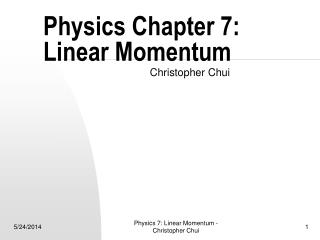DownloadDownload PresentationPhysics Chapter 7: Linear Momentum

# Physics Chapter 7: Linear Momentum

Download Presentation## Physics Chapter 7: Linear Momentum

- - - - - - - - - - - - - - - - - - - - - - - - - - - E N D - - - - - - - - - - - - - - - - - - - - - - - - - - -
##### Presentation Transcript

1. Physics Chapter 7: Linear Momentum Christopher Chui Physics 7: Linear Momentum - Christopher Chui

2. Linear Momentum • Linear momentum, p = mv • Newton’s 2nd law: The rate of change of momentum of a body is equal to the net force applied to it. SF = Dp / Dt • Conservation of momentum for two bodies: momentum before = momentum after • The total momentum of an isolated system of bodies remains constant • Impulse = FDt = Dp = change in momentum Physics 7: Linear Momentum - Christopher Chui

3. Conservation of Energy & Momentum in Collisions • In an elastic collision, KE is conserved • In inelastic collisions, friction and heat are generated • If two objects stick together after collision, the collision is inelastic • Inelastic collisions are not conservative Physics 7: Linear Momentum - Christopher Chui

4. Problem Solving: Momentum Conservation and Collisions • Be sure no external force acts on the system • Draw a diagram of the initial situation. Do the same after the collision • Choose a coordinate system and label + or – • Write momentum conservation equations • If elastic, conservation of KE also applies: total initial KE = total final KE • Solve the unknowns using simple algebra Physics 7: Linear Momentum - Christopher Chui

5. Center of Mass (CM) • CM is the point where the mass is centered • In motion, an object moves wrt the CM • The general motion of an extended body is the sum of the translational motion of the CM, and rotational, vibrational, or other types of motion about the CM • For most purposes, CM is the same as CG • CM can be outside the body • The CM of a system of particles with total mass M moves like a single particle of mass M acted upon by the same net external force Physics 7: Linear Momentum - Christopher Chui